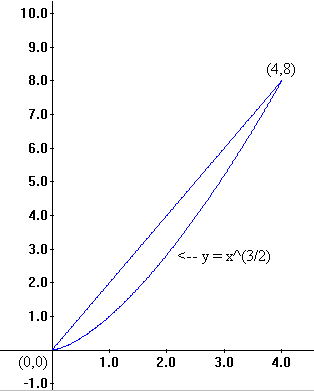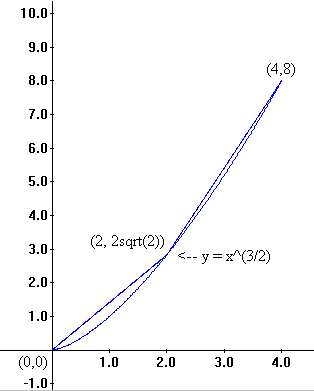Chapter VIII.B Arc Length
VIII.B. More Applications of Integration

Feb., 2006: This page now requires Internet Explorer 6+MathPlayer or Mozilla/Firefox/Netscape 7+.

Measuring Arc Length

In this section we will look two related approaches using integration to measure the length of a curve in the plane. In particular the curves in our discussion are the graphs of functions (either explicit or implicit) with continuous first derivatives.

In our first approach the key tool for finding the arc length will be the approximation of this length as a sum of line segment lengths determined by the given curve. These estimating sums will have a limiting value which by definition is a definite integral.

Our second approach to the arc length question uses an estimate of the rate at which the arc length is changing as a function of one variable. In other words, imagine an object moving along the curve with its first coordinate changing at a unit speed. We estimate the speed of the object moving on the curve. This estimate also uses a line segment length to approximate the change in position along the arc.

Arc Length as the Limit of Sums

Perhaps the easiest way to estimate the distance between two points along the curve `Y = f (X)` is to ignore the curve and find the distance between the two points along a line segment between them . One might say we use the distance between them ``as the crow flies.'' If the points have coordinates `(x,y)` and
`(x+Delta x ,y + Delta y)` then the distance between them is

`sqrt{Delta x ^2 + Delta y ^2 } = Delta x sqrt{ 1 + ({Delta y}/ {Delta x})^2}`.

When `Delta x` is close to 0 we know that `{Delta y} /{Delta x}` approaches `f '(x)`, and therefore `sqrt{ 1 + ({Delta y}/ {Delta x})^2}`  approaches
`sqrt {1 + (f'(x))^2}`. Now we only need to consider how we accumulate these estimates to approximate the length of the arc.

Keep in mind the definition of the definite integral, namely:

`int _a^b P(x) dx = lim _{Delta x -> 0} Sigma_{k=0}^{k=n-1} P(x_k) Delta x`.

So we continue to estimate the length of the arc of the graph of the function between `(a, f (a))` and `(b, f (b))`. Partition the interval `[a,b]`, make the same type of estimate for each subinterval as above using`sqrt{Delta x_k ^2 + Delta y_k ^2 } = Delta x_k sqrt{ 1 + ({Delta y_k}/ {Delta x_k})^2}`  so the arc length is approximated by

`Sigma _{k=0}^{n-1}  sqrt{ 1 + ({Delta y_k}/ {Delta x_k})^2} Delta x_k`.
Now to find the arc's length consider `Delta x` close to 0 and the estimates approach the arc length while they also approach (by definition)
`int _a^b sqrt{1+ (f'(x))^2} dx ` .

Thus the arc length of the graph of the function between `(a,f (a))` and `(b,f (b))` is `int _a^b sqrt{1+ (f'(x))^2} dx `.

Here's an example to make this discussion more concrete.

 Example VIII.B.1. a) Estimate the arc length of the curve that is the graph of  `f(x) = x^{3/2}` between the points (0,0) and (4,8). b) Express the arc length of the curve in a) as a definite integral and explain how this integral is related to the estimate in part a). Solution. a)  First graph the curve under discussion and note that the simplest estimate is the distance between the two endpoints, namely, `sqrt{4^2+(4^3/2)^2} = sqrt{80} = 4 sqrt{5}` or approximately 8.94427191. As a next estimate we might cut the interval [0,8] in half and estimate the arclength by adding the lengths of the two line segments between (0,0), (2,`2sqrt{2}`) and (4,8). This gives the estimate of `sqrt{4+8} + sqrt{ 4+72-32sqrt{2}}` or approximately 9.008933984. Of course a finer partition of the interval and a correspondingly larger number of line segments would give a better estimate of the arc length. Now if we let `Delta x = 4/n`, `x_k = {4k}/n`, and `Delta y_k = x_{k+1}^{3/ 2} - x_k^{3 / 2}`, then the estimate for the arc length is given by `Sigma _{k=0}^{n-1}  sqrt{ 1 + ({Delta y_k}/ {Delta x})^2} Delta x` Larger values for n will give a better estimate for the arc length as Figure VIII.B.1 illustrates. Using a spreadsheet with n = 10 we find an estimate of approximately 9.065690735. [You should check this with a spreadsheet as well]. b) The arc length in part a) has been estimated with a sum that estimates a definite integral, namely `int_0^4 sqrt{1 + (f'(x))^2} dx = int_0^4 sqrt{1 + (3/2 sqrt{x})^2} dx = int_0^4 sqrt{1 +9/4 x} dx ~~ 9.0734`.Generalization Using Riemann Sums. The work in the last example can be generalized and made more rigorous in the estimation without much difficulty by using Riemann sums to find the definite integral. In a general situation the arc will be the graph of a function f between the points `(a,f(a))` and `(b,f(b))` where `f` has a continous derivative function `f'` on `[a,b]`. We partition the interval `[a,b]` into `n` pieces of length `Delta x = (b-a)/n` with `x_k = a + k Delta x` , `Delta y_k = f (x_{k+1} ) - f (x_k)`. We consider the line segment length `Delta s_k` for each subinterval `[x_k,x_{k+1} ]`. `Delta s_k = sqrt{ (Delta x)^2  +  (Delta  y_k)^2} = sqrt{ 1 +  ({Delta y_k}/ {Delta x })^2} Delta x`.
Using the Mean Value Theorem for `f` on each of these subintervals gives points `z_k in [x_k ,x_{k+1}]` and we have

`{Delta y_k}/{Delta x} = f '(z_k).
The estimating sum of these lengths for the individual line segments is given by `Sigma_1^n Delta s_k = Sigma_ 1^n sqrt{ 1 +  ({Delta y_k}/ {Delta x })^2} Delta x =Sigma_1^n sqrt{ 1 +  (f'(z_k))^2} Delta x `. As `n->oo` , these sums give better estimates for the arc length of the curve, but they also approach `int _a^b sqrt{1+ (f'(x))^2} dx`   by the definition of the (Riemann) definite integral. Therefore the arc length of the curve is given by `int _a^b sqrt{1+ (f'(x))^2} dx `.
Length and Speed: Differential Equations Again!

Imagine in the example we considered for arc length that we are moving a light along the X-axis which is shining on the point vertically above it on the graph of `Y=X^{3/2}`. The light is moving at a constant unit rate along the X-axis . How fast is the light image moving along the curve? This question can be addressed in two different ways, one using the arc length formula we just discovered and the other leading to a different way to find the arc length formula.

From our previous work the length of arc that the light has travelled from when it

was at `(a, a ^{3/2} )` to when it is at `(t, t^{3/2} )` is s(t) = `int _1^t sqrt{1 +9/4 x} dx`.
Now a simple application of the derivative form of the Fundamental Theorem of Calculus shows that `s'(t) = sqrt{1 +9/4 t}`.

In general the same result is true: The speed of an object moving along a curve which is the graph of `Y = f(X)` and with its position determined by `s(t)` where
`s(t) =  int _a^t sqrt{1+ (f'(x))^2}` dx  is given by `s'(t) = sqrt{1+ (f'(t))^2}`.
This establishes that the arc length is the solution to the differential equation

`s'(t) = sqrt{1+ (f'(t))^2}`, where `s(a) = 0` .

It is possible to arrive at this differential equation directly from the knowledge of the function `f`. Consider `s(t)` at the distance travelled on the arc at time `t`. Then `s(t+Delta t)-s(t)` is the distance travelled along the arc between time `t` and `t + Delta t`. When `Delta t` is close to zero, this distance can be estimated by the distance between the points `(t,f(t))` and `(t+Delta t, f(t+Delta t) )`. It should not be hard to see at this stage that this distance is
`sqrt{(Delta t)^2 + (f(t+ Delta t) - f(t))^2 }=sqrt{(Delta t)^2 + (f'(z) Delta t)^2 }` where `z ` is between `t` and `t + Delta t`. As `Delta t -> 0` we see that
`{s(t + Delta t) - s(t)}/{Delta t } =  sqrt{ (Delta t)^2 + (f(t+ Delta t) - f(t))^2 }/{Delta t} = {Delta t sqrt{1 +(f'(z ))^2 }}/{Delta t}->sqrt{1+ (f'(t))^2}`.
Therefore `s'(t) = sqrt{1+ (f'(t))^2}`.

Optional: Arc Length for Curves Determined by Motion in the Plane
Suppose the position of an object moving in the plane determines a curve so that at time `t` the position of the object on the curve has coordinates `(x,y) =(x(t),y(t))` where `x` and `y` are continuously differentiable functions of the variable `t`. To find the length of the arc of this curve between time `t = a` and `t = b`  we can use an analysis by estimation analogous with that done for the graph of a function. For a small interval of time we can estimate the length with the distance between the end points of the curve:
`Delta x = x(b) - x(a)` and `Delta y = y(b)-y(a)` so the length is estimated by `sqrt{(Delta x) ^2 + (Delta y) ^2}` `=sqrt{({Delta x}/{Delta t}) ^2 + ({Delta y}/{Delta t})^2}Delta t`.

Partition the time interval `[a,b]` with  resulting cuts on the curve at points `(x(t_k), y(t_k))`. We estimate the length of each section of the curve as we did for the single interval of time:`Deltax_k = x(t_{k+1}) - x(t_k)` and `Delta y_k = y(t_{k+1})-y(t_k)` so the length is estimated by `sqrt{(Delta x_k)^2+(Delta y_k)^2}=sqrt{({Delta x_k}/{Delta t})^2+({Delta y_k}/{Delta t})^2}Delta t` and the total arc length is estimated by the sum: `Sigma _0^{n-1} sqrt{({Delta x_k}/{Delta t}) ^2 + ({Delta y_k}/{Delta t})^2}Delta t`.
Now as `n->oo` these sums estimate the arc length for the curve and an integral as well- namely: `int_{t=a}^{t=b} sqrt{({dx}/{dt}) ^2 + ({dy}/{dt}) ^2 }dt.`
So the arc length for this curve is `int_{t=a}^{t=b} sqrt{({dx}/{dt})^2 + ({dy}/{dt}) ^2 } dt = int_{t=a}^{t=b} sqrt{(x'(t)) ^2 + (y'(t)) ^2} dt.`
Note: The previous formula for arclength for the graph of a function `f` can be found in this new formula by using `x(t) = t` and `y(t)= f(t)`.

Example VIII.B.2 Suppose `x(t) = 3cos(t)` and `y(t) = 3sin(t)` with `0=< t =< 2pi`. Then the curve is a circle of radius 3 and the arclength is the circumference.
Using  the formula just derived- the arclength is given by  `int_0^{2pi} sqrt { 9sin^2(t) + 9cos^2(t)} dt = int_0^{2pi}3 dt =  6pi.`

Summary

If `f` is a function with a continuous derivative on the interval `[a,b]` then the length of the arc of the graph of `f` over the interval `[a,b]` is given by `int _a^b sqrt{1+ (f'(x))^2} dx `.

If `s(t)` is the length of the arc of the graph of `f` over the interval `[a,t]`, then   `s'(t) = sqrt{1+ (f'(t))^2}` , and `s(t) = int _a^t sqrt{1+ (f'(x))^2} dx `.

Exercises.

In each of the following problems
a) sketch a graph of the curve described,
b) use four chords to estimate the arc length of the curve,
c) express the arc length of the curve using a definite integral,
d) when possible find the exact arc length of the curve described.

1. The graph of Y 2 = X3 between (0,0) and (1,1).

2. The graph of Y 2 = X3 between (0,0) and (4,8).

3. The graph of Y = X 2 between (0,0) and (1,1).

4. The graph of Y = X 2 between (0,0) and (2,4).

5. The graph of Y = X 2 between (-1,1) and (1,1).

6. The graph of Y 2 = X between (0,0) and (1,1).

7. The graph of Y 2 = X between (0,0) and (4,2).

8. The graph of Y = 1/X between (1,1) and (2,1/2).

9. The graph of Y = 1/X between (1,1) and (3,1/3).